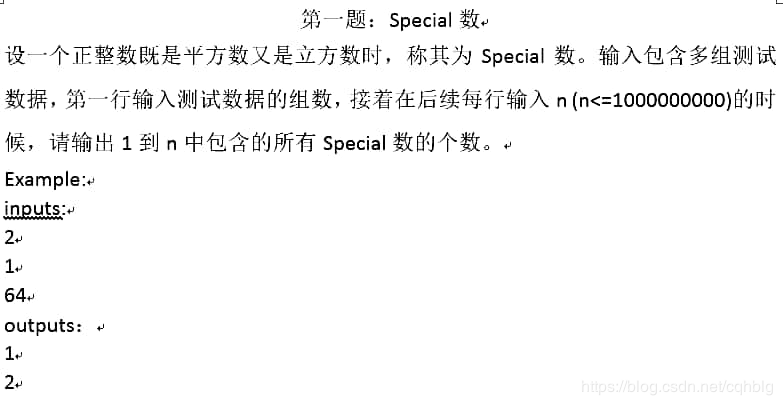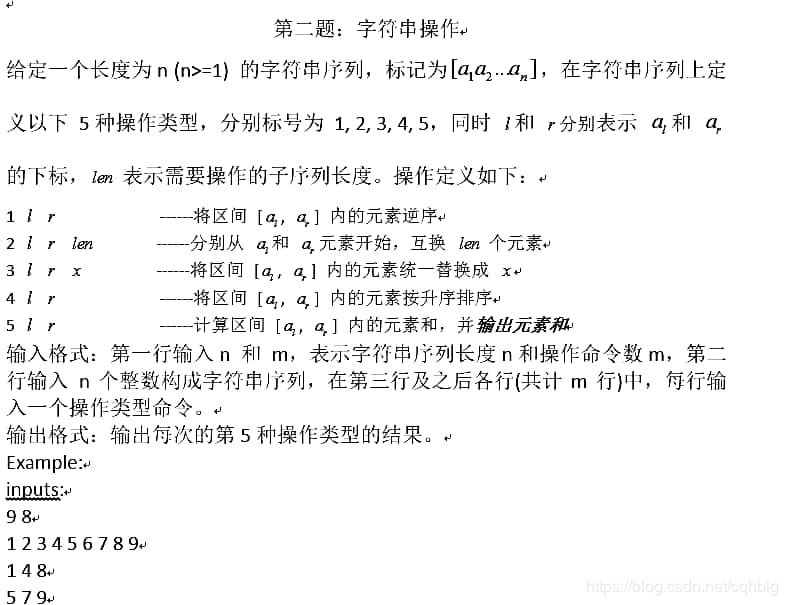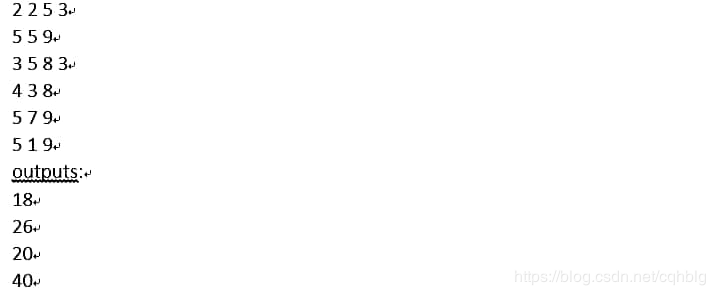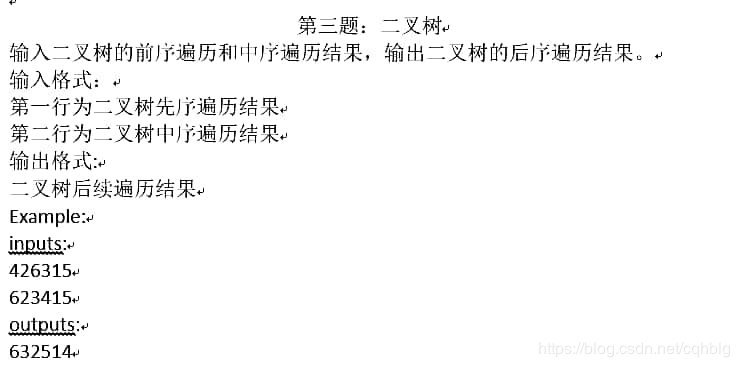• 这里我汇总了绝大部分北邮BUPT OJ 上的中文题，中文题也就都是往年题。有从10年开始到14年的所有机试往年题。我的博客里有上述文档里所有的题。谁知道怎么降低下载积分？现在看不到这个选项了
• 计算机 原博题目搬运 求10^9以内6次方数的个数 #include <bits/stdc++.h> using namespace std; #define max 1000000000 int main(){ int k,n; long special; int size=0; for(int i=1;......
计算机
原博题目搬运求10^9以内6次方数的个数
#include <bits/stdc++.h>
using namespace std;
#define max 1000000000
int main(){
int k,n;
long special;
int size=0;
for(int i=1;i<=30;i++)
if(pow(i,6)<=max) special[size++]=pow(i,6);
char str,op,subs;
for(scanf("%d",&k);k--;){
scanf("%d",&n);
int i=0;
for(;special[i]<=n;i++);
printf("%d\n",i);
}
return 0;
}9 8 1 2 3 4 5 6 7 8 9 1 4 8 5 7 9 2 2 5 3 5 5 9 3 5 8 3 4 3 8 5 7 9 5 1 9

/*
9 8
1 2 3 4 5 6 7 8 9
1 4 8
1 2 3 8 7 6 5 4 9
5 7 9
18
1 2 3 8 7 6 5 4 9
2 2 5 3
1 7 6 5 2 3 8 4 9
5 5 9
26
1 7 6 5 2 3 8 4 9
3 5 8 3
1 7 6 5 3 3 3 3 9
4 3 8
1 7 3 3 3 3 5 6 9
5 7 9
20
1 7 3 3 3 3 5 6 9
5 1 9
40
1 7 3 3 3 3 5 6 9
*/
**#include <bits/stdc++.h>
using namespace std;
int main(){
int n,m,num;
scanf("%d%d",&n,&m);
for(int i=1;i<=n;i++)
scanf("%d",&num[i]);
while(m--){
int op,l,r,lorx;
scanf("%d%d%d",&op,&l,&r);
switch(op){
case 1:
printf("%d %d\n",num[l],num[r]);
for(int i=l;i<(l+r+1)/2;i++)
swap(num[i],num[r-i+l]);
for(int i=1;i<=n;i++)
printf("%d ",num[i]);
printf("\n");
break;
case 2:
printf("%d %d\n",num[l],num[r]);
scanf("%d",&lorx);
int tmp;
for(int i=0;i<lorx;i++)
swap(num[l+i],num[i+r]);
for(int i=1;i<=n;i++)
printf("%d ",num[i]);
printf("\n");
break;
case 3:
printf("%d %d\n",num[l],num[r]);
scanf("%d",&lorx);
for(int i=l;i<=r;i++)
num[i]=lorx;
for(int i=1;i<=n;i++)
printf("%d ",num[i]);
printf("\n");
break;
case 4:
printf("%d %d\n",num[l],num[r]);
sort(num+l,num+r+1);
for(int i=1;i<=n;i++)
printf("%d ",num[i]);
printf("\n");
break;
case 5:
printf("%d %d\n",num[l],num[r]);
int res=0;
for(int i=l;i<=r;i++)
res+=num[i];
printf("%d\n",res);
for(int i=1;i<=n;i++)
printf("%d ",num[i]);
printf("\n");
break;
}
}
return 0;
}**#include <bits/stdc++.h>
using namespace std;
struct node{
char data;
node* l;
node* r;
node(char data):data(data),l(NULL),r(NULL){}
};
node* creat(string pre,string in){
if(pre.size()==0) return NULL;
node* x=new node(pre);
int idx=in.find(pre);
x->l=creat(pre.substr(1,idx),in.substr(0,idx));
x->r=creat(pre.substr(idx+1),in.substr(idx+1));
return x;
}
void postorder(node* t){
if(t!=NULL){
postorder(t->l);
postorder(t->r);
printf("%c",t->data);
}
}
int main(){
char po,io;
scanf("%s\n%s",po,io);
string pre(po),in(io);
node* x=creat(pre,in);
postorder(x);
printf("\n");
return 0;
}

展开全文• 北邮考研复试 | 机试 | 2014年北邮网研院机试（下午） 计算机院 北邮考研复试 | 机试 | 2014年北邮计算机院机试（下午） 北邮考研复试 | 机试 | 2014年北邮计算机院机试（上午） 机试常用的数据结构/模板/基础...
目录
网研院
北邮考研复试 | 机试 | 2014年北邮网研院机试（下午）
计算机院
北邮考研复试 | 机试 | 2014年北邮计算机院机试（下午）
北邮考研复试 | 机试 | 2014年北邮计算机院机试（上午）
机试常用的数据结构/模板/基础知识
C/C++ | STL | set
C/C++ | STL vector
C | C++ | STL map
C/C++ | 全排列 | next_permutation | prev_permutation | 常规全排列
C/C++ | 字符串数组的分割和string的分割 | strtok()函数 | find()和substr()函数
C | struct结构体 | 结构体数组 | typedef struct | 结构体初始化 | 结构体构造函数
C/C++ | STL | 大顶堆 | 小顶堆 | std::priority_queue

正在慢慢填坑中！！！
下面是我自己打印版的链接（里面有往年题大概几十页）。
百度网盘
链接：https://pan.baidu.com/s/1YcVb6rFTFXKrZlQmrjr7Cw  提取码：r7nc
4.11号更新 ： 对不起，我最近在忙毕设，忙完之后我会慢慢补全所有的题，包括近几年的回忆版题。

网研院
北邮考研复试 | 机试 | 2014年北邮网研院机试（下午）
https://blog.csdn.net/stone_fall/article/details/89014007

计算机院
北邮考研复试 | 机试 | 2014年北邮计算机院机试（下午）
https://blog.csdn.net/stone_fall/article/details/88930792
北邮考研复试 | 机试 | 2014年北邮计算机院机试（上午）
https://blog.csdn.net/stone_fall/article/details/88963703

机试常用的数据结构/模板/基础知识
C/C++ | STL | set
https://blog.csdn.net/stone_fall/article/details/88823980
C/C++ | STL vector
https://blog.csdn.net/stone_fall/article/details/88757543
C | C++ | STL map
https://blog.csdn.net/stone_fall/article/details/88669763
C/C++ | 全排列 | next_permutation | prev_permutation | 常规全排列
https://blog.csdn.net/stone_fall/article/details/88670531
C/C++ | 字符串数组的分割和string的分割 | strtok()函数 | find()和substr()函数
https://blog.csdn.net/stone_fall/article/details/88640469
C | struct结构体 | 结构体数组 | typedef struct | 结构体初始化 | 结构体构造函数
https://blog.csdn.net/stone_fall/article/details/88363197
C/C++ | STL | 大顶堆 | 小顶堆 | std::priority_queue
https://blog.csdn.net/stone_fall/article/details/89010656 
展开全文• 打牌问题题目描述输入描述输出描述输入示例输出示例总结 题目描述 牌只有1到9，手里拿着已经排好序的牌a，对方出牌b，用程序判断手中牌是否能够压过对方出牌。 规则：出牌牌型有5种 一张 如4 则5…9可压过 两...


打牌问题
题目描述输入描述输出描述输入示例输出示例
总结

题目描述

牌只有1到9，手里拿着已经排好序的牌a，对方出牌b，用程序判断手中牌是否能够压过对方出牌。 规则：出牌牌型有5种 一张 如4 则5…9可压过 两张 如44 则55，66，77，…，99可压过 三张 如444 规则如 四张 如4444 规则如 五张 牌型只有12345 23456 34567 45678 56789五个，后面的比前面的均大。

输入描述

输入有多组数据。 每组输入两个字符串(字符串大小不超过100)a，b。a字符串代表手中牌，b字符串代表处的牌。

输出描述

压过输出YES 否则NO。

输入示例

12233445566677 33

输出示例

YES


public class Main{
public static void main(String[] args){
Scanner in = new Scanner(System.in);
while(in.hasNextLine()){
String a = in.nextLine();
String b = in.nextLine();
int[] count = new int;
int flag=0;
for(int i=0;i<a.length();i++){
count[a.charAt(i)-'0']++;
}
if(b.length()>0&&b.length()<=4){
for(int i=b.charAt(0)-'0'+1;i<10;i++){
if(count[i]>=b.length()){
flag=1;
}
}
}else if(b.length()==5){
for(int i=b.charAt(0)-'0'+1;i<=5;i++){
if(count[i]>0&&count[i+1]>0&&count[i+2]>0&&count[i+3]>0&&count[i+4]>0){
flag=1;
}
}
}
if(flag==1){
System.out.println("YES");
}else if(flag==0){
System.out.println("NO");
}
}
}
}

总结

开始总想着把所有的牌型进行罗列出来，其实用循环有更简洁的做法

展开全文• 如果长度超过32位就不用前导0 题目来源 北京邮电大学2019年机试题 真题链接在此 思路：就是从低位向高位进行处理，然后注意进位即可。坑：11111111111111111111111111111111的结果是...
A、 二进制

Time Limit: 1000 ms Memory Limit: 256 mb 有一个长度为32位的二进制数X，对其进行X+1,X+3 操作，并输出。注意不能忽略前导0

输入输出格式 输入描述: 第一行，一个整数T,代表测试数据组数。 接着T行，输入32为二进制数 输出描述: 对每组测试数据，输出两行，第一行为X+1,第二行为X+3.

输入输出样例 输入样例#: 2 00000000000000000000000000000000 00000000000000000000000000000001 输出样例#: 00000000000000000000000000000001 00000000000000000000000000000011 00000000000000000000000000000010 00000000000000000000000000000100 提示 如果长度超过32位就不用前导0

题目来源 北京邮电大学2019年机试题 真题链接在此

思路：就是从低位向高位进行处理，然后注意进位即可。坑：11111111111111111111111111111111的结果是100000000000000000000000000000000（输出33位）和100000000000000000000000000000010（输出33位）
#include<iostream>
#include<algorithm>
#include<cmath>
#include<cstring>
using namespace std;
int main(){
int t;
cin>>t;
string h;
for(int i=1;i<=t;i++){
cin>>h;
int k=1;
int mark=0;
for(int j=31;j>=0;j--){
int ll=h[j]-'0'+k;
if(ll==2){
if(j==0)
mark=1;
k=1;
h[j]='0';
}
else{
h[j]='0'+ll;
k=0;
}
}
if(mark==0)
cout<<h<<endl;
else{
cout<<1;
for(int u=0;u<32;u++)
cout<<h[u];
cout<<endl;
}
k=1;
for(int j=30;j>=0;j--){
int ll=h[j]-'0'+k;
if(ll==2){
k=1;
h[j]='0';
}
else{
h[j]='0'+ll;
k=0;
}
}
if(mark==0)
cout<<h<<endl;
else{
cout<<1;
for(int u=0;u<32;u++)
cout<<h[u];
cout<<endl;
}
}
return 0;
}

B、二叉树

Time Limit: 1000 ms Memory Limit: 256 mb 对二叉树，计算任意两个结点的最短路径长度。

输入描述: 第一行输入测试数据组数 T 第二行输入 n , m 。 n 代表输入的数据组数， m 代表要查询的数据组数。 接下来 n 行，每行输入两个数，代表1~ n 结点的孩子结点，如果没有孩子结点则输入-1，根节点为1。 接下来 m 行，每行输入两个数，代表要查询的两个结点。 输出描述: 每组测试数据输出 m 行，代表查询的两个结点之间的最短路径长度。

输入输出样例 输入样例#: 1 8 4 2 3 4 5 6 -1 -1 -1 -1 7 -1 -1 8 -1 -1 -1 1 6 4 6 4 5 8 1 输出样例#: 2 4 2 4

题目来源 北京邮电大学2019年机试题 真题链接在此

思路：用len数组记录当前节点的深度，findRoot函数是记录当前节点的父亲节点。我们可以让两个目标节点同时向上找第一个相同的祖先节点，当找到第一个相同的祖先节点时，第一个节点到祖先节点的距离+第二个节点到祖先节点的距离就是两个节点之间的最小距离。我们每一次找祖先节点的时候都是首先操作在树中更深的节点，因为有可能另一个节点就是当前节点的祖先节点，为了避免错过这个节点所以不可以两边的节点同时向上找共同的祖先节点。
#include<iostream>
#include<algorithm>
#include<cmath>
#include<cstring>
using namespace std;
int father;
int len;
int dis;
void findRoot(int a,int b){
if(a==b)
return;
dis++;
if(len[a]>=len[b])
findRoot(father[a],b);
else if(len[a]<len[b])
findRoot(a,father[b]);
return;
}
int main(){
int t;
cin>>t;
while(t--){
memset(father,0,sizeof(father));
memset(len,0,sizeof(len));
father=-1;
len=1;
int n,k;
cin>>n>>k;
for(int i=1;i<=n;i++){
int a,b;
cin>>a>>b;
if(a!=-1){
father[a]=i;
len[a]=len[i]+1;
}
if(b!=-1){
father[b]=i;
len[b]=len[i]+1;
}
}
for(int i=0;i<k;i++){
int a,b;
cin>>a>>b;
dis=0;
findRoot(a,b);
cout<<dis<<endl;
}
}
return 0;
}

C、最短路径

Time Limit: 1000 ms Memory Limit: 256 mb 有n（n<=50）个城市，保证每个城市与其他城市之间必然有连接，但是两个城市之间会存在多条道路，输入道路连接的两个城市号及道路长度。同时在夜晚，某些道路会封路。请输出在白天和夜晚从城市1到城市n之间的最短路径

输入描述: 先输入T，表示有T组数据 再输入n，m，k，n表示有n个城市，表示总共有m条边，k表示在夜晚有k条路封路 接下来m行，每行输入三个数x,y，z,代表城市x和城市y之间的路径距离 最后一行k个数，代表晚上关闭的线路序号（线路序号指的是1~m） 输出描述: 每组数据输出两行，分别代表白天和黑夜，城市1到n的最短路径

输入样例#: 1 4 4 1 1 2 1 2 3 1 3 4 1 1 4 1 4 输出样例#: 1 3

题目来源 北京邮电大学2019年机试题 真题链接在此

思路：就是最普通的单源最短路，当然不想写Dijkstra的也可以直接上Floyd，不会超时。除了最短路的板子，就是一开始对数据的预处理了，用Floyd的话，邻接矩阵是一个对阵矩阵，一开始令所有元素均为无穷大，然后读入m条边，并修改邻接矩阵中对应的值，注意因为是对称矩阵所以一次性修改两个值，坑点1：因为两个城市之间不止一条路，所以要记录两个城市之间距离最小的那条，然后跑一次最短路即可。接下来是晚间，因为晚上有的路不能走，所以我们要令相对应的路的值为无穷大。坑点2：因为我们之前在第一次最短路操作时已经对邻接矩阵中的很多值进行了修改，再进行一次新的最短路，应该先令邻接矩阵的值都为无穷大，然后再一次遍历那m条边，操作和第一次一样，但是一些路已经是无穷大了即晚间不可通行，这里不用特殊处理这些路（坑点2我自己踩了，debug到头秃，值得注意别粗心）。总体思路就是两次最短路，但要注意数据预处理。
不知道最短路怎么写的，可移步我的另一篇博客，里面有多种最短路的算法 最短路的各种算法小节链接在此

Floyd版本

#include<iostream>
using namespace std;
struct node {
int x,y,z;
};
int main()
{
int t;
cin>>t;
for(int i=1; i<=t; i++) {
int h;
node p;
int n,m,kk;
cin>>n>>m>>kk;
int num1;
for(int j=1; j<=n; j++){
for(int k=1; k<=n; k++){
h[j][k]=99999999;
h[k][j]=99999999;
}
}
for(int j=1; j<=m; j++) {
cin>>p[j].x>>p[j].y>>p[j].z;
int ll=h[p[j].x][p[j].y];
if(p[j].z<ll) {
h[p[j].x][p[j].y]=p[j].z;
h[p[j].y][p[j].x]=p[j].z;
}
}
for(int k1=1;k1<=n;k1++)
for(int i1=1;i1<=n;i1++)
for(int j1=1;j1<=n;j1++)
if(h[i1][j1]>h[i1][k1]+h[k1][j1])
h[i1][j1]=h[i1][k1]+h[k1][j1];
num1=h[n];
for(int j=1; j<=kk; j++) {
int l;
cin>>l;
p[l].z=99999999;
h[p[l].x][p[l].y]=99999999;
h[p[l].y][p[l].x]=99999999;
}
//h数组全都要置为99999999因为第一次的floyed已经使得h中很多数据都改变了！！！
for(int j=1;j<=n;j++){
for(int k=1;k<=n;k++){
h[j][k]=99999999;
h[k][j]=99999999;
}
}
for(int j=1;j<=m;j++){
if(h[p[j].x][p[j].y]>p[j].z){
h[p[j].x][p[j].y]=p[j].z;
h[p[j].y][p[j].x]=p[j].z;
}
}
for(int k1=1;k1<=n;k1++)
for(int i1=1;i1<=n;i1++)
for(int j1=1;j1<=n;j1++)
if(h[i1][j1]>h[i1][k1]+h[k1][j1])
h[i1][j1]=h[i1][k1]+h[k1][j1];
cout<<num1<<endl<<h[n]<<endl;
}
return 0;
}


Dijkstra版本

#include<iostream>
using namespace std;
struct node {
int x,y,z;
};
int main()
{
int t;
cin>>t;
for(int i=1; i<=t; i++) {
int h;
node p;
int n,m,kk;
cin>>n>>m>>kk;
int num1;
for(int j=1; j<=n; j++){
for(int k=1; k<=n; k++){
h[j][k]=99999999;
h[k][j]=99999999;
}
}
for(int j=1; j<=m; j++) {
cin>>p[j].x>>p[j].y>>p[j].z;
int ll=h[p[j].x][p[j].y];
if(p[j].z<ll) {
h[p[j].x][p[j].y]=p[j].z;
h[p[j].y][p[j].x]=p[j].z;
}
}
int dis;
int book;
for(int j=1; j<=n; j++)
dis[j]=h[j];
for(int j=1; j<=n; j++)
book[j]=0;
book=1;
int min;
int u,v;
for(int j=1; j<=n-1; j++) {
min=99999999;
for(int k=1; k<=n; k++) {
if(book[k]==0&&dis[k]<min) {
min=dis[k];
u=k;
}
}
book[u]=1;
for(v=1; v<=n; v++) {
if(h[u][v]<99999999) {
if(dis[v]>dis[u]+h[u][v])
dis[v]=dis[u]+h[u][v];
}
}
}
num1=dis[n];
for(int j=1; j<=kk; j++) {
int l;
cin>>l;
p[l].z=99999999;
}
for(int j=1;j<=n;j++){
for(int k=1;k<=n;k++){
h[j][k]=99999999;
h[k][j]=99999999;
}
}
for(int j=1;j<=m;j++){
if(h[p[j].x][p[j].y]>p[j].z){
h[p[j].x][p[j].y]=p[j].z;
h[p[j].y][p[j].x]=p[j].z;
}
}
for(int j=1; j<=n; j++)
dis[j]=h[j];
for(int j=1; j<=n; j++)
book[j]=0;
book=1;
for(int j=1; j<=n-1; j++) {
min=99999999;
for(int k=1; k<=n; k++) {
if(book[k]==0&&dis[k]<min) {
min=dis[k];
u=k;
}
}
book[u]=1;
for(v=1; v<=n; v++) {
if(h[u][v]<99999999) {
if(dis[v]>dis[u]+h[u][v])
dis[v]=dis[u]+h[u][v];
}
}
}
cout<<num1<<endl<<dis[n]<<endl;
}
return 0;
}

D、方块阵

Time Limit: 1000 ms Memory Limit: 256 mb 给出一张从原图片中沿横纵向剪切后的图片，判断原图片中nn（n>=1）矩阵的大小 （原图片肯定存在该nn的矩阵，且唯一） 举例说明 如果原图片是这样： …###… …###… …###… … … 剪切后的图片可能是： 1）不变 …###… …###… …###… … … 2） ##… ##… ##… … … 所以一个原图片可能对应很多剪切后的图片

输入描述: 输入剪辑后的图片，长宽不会超过100 其中‘.’表示空白，’#'表示图片中矩阵的内容 输出描述: 输出原图片中最小n*n矩阵的大小，即n的值，如果不存在则输出-1

输入样例#: ##… ##… ##… … … 输出样例#: 3

题目来源 北京邮电大学2019年机试题 真题链接在此

思路：就是读入当前的字符矩阵，找出以行为单位找出含#最多的行有多少个#，然后以列为单位找出含#最多的列有多少个#，然后取两者的最大值即为n的值的大小，如果两者均为0，则可知找不到输出-1即可。关于输入的一个小问题，我们在DEV类似的编译器上运行该程序，可能会出现一直需要输入的情况，因为whlie中并没有退出的条件，因为题目中也没有给出输入什么时候可以判断结束，读者可以自行写一个退出while循环的条件，比如给的样例一共5行，我们可以令while循环执行5次退出即可，注意这只是调试的时候可以使用，提交的时候可以不用管while什么时候可以退出，直接交上就行，本人已经AC。
#include<iostream>
#include<cstdio>
#include<algorithm>
#include<cstring>
#include<cmath>
using namespace std;
int main(){
string h2;
int num1=0;
int num2=0;
char h;
int a=0;
int l;
while(cin>>h2){
l=h2.size();
for(int i=0;i<l;i++)
h[a][i]=h2[i];
a++;
}
for(int i=0;i<a;i++){
int n=0;
for(int j=0;j<l;j++){
if(h[i][j]=='#')
n++;
}
if(n>num1)
num1=n;
}
for(int j=0;j<l;j++){
int n=0;
for(int i=0;i<a;i++){
if(h[i][j]=='#')
n++;
}
if(n>num2)
num2=n;
}
if(num1==0&&num2==0)
cout<<"-1\n";
else{
if(num1>num2)
cout<<num1<<endl;
else
cout<<num2<<endl;
}
return 0;
}

展开全文• 字符串转换 时间限制 1000 ms 内存限制 65536 KB 题目描述 我们将仅由若干个同一小写字母构成的字符串称之为简单串，例如"aaaa"是一个简单串，而"abcd"则不是简单串。现在给你一个仅由小写...
• 代码量很大，机试时候肯定做不出来，最后几天了，花时间准备笔试，代码基本都是抄一抄看看思路 代码参考： https://blog.csdn.net/u012963208/article/details/64165144 #include #define For(i,start,...图的遍历
• 一.比较奇偶数个数 5 1 5 2 4 3 #include <bits/stdc++.h> using namespace std; int main(){ int n,num; while(scanf("%d",&n)!=EOF){ int even=0,odd=0;... scanf("%d",&...
• 二叉树的层数 时间限制 1000 ms 内存限制 65536 KB 题目描述 老师有一个问题想考考mabo，但是mabo不会，所以想请你来帮帮忙。 问题如下： ...一个节点是先添加左节点后添加右节点，即添加顺序与输入顺序一致。...二叉树层次遍历
• 统计节点个数 时间限制 1000 ms 内存限制 65536 KB 题目描述 给出一棵有向树，一共有N(1<N≤1000)个节点，如果一个节点的度（入度+出度）不小于它所有儿子以及它父亲的度(如果存在父亲或儿子)，那么我们称这个...
• 一、转自大佬：https://blog.csdn.net/TQCAI666/article/details/85600413，只为学习，侵删 二、目录： 基本操作 1 C++ 常用头文件和宏定义 1 输入输出 2 二分 3 排序 3 图论 4 基本数据结构 4 ...线...
• 84. Single Number 时间限制1000 ms内存限制65536 KB 题目描述 Given an array withNintegers where all elements appear three times except for one. Find out the one which appears ... 输入格式 ...Several te...
• 题目：设一个正整数既是平方数又是立方数时，称其为special数。 输入包含多组测试数据，第一行输入测试数据的组数，接着在后续每行输入n（n<= 1000000000）的时候， 请输出1到n中包含的所有special数的个数。...
• 这道题，我本来想用上道oj上图像翻转的规律，结果并不成功，最后用了下面的本办法。 #include<bits/stdc++.h> using namespace std; int buf; int ans; int main(){ ...j&l...
• 模拟哈夫曼树的总权值的计算过程，不用真的建树。 #include<bits/stdc++.h> using namespace std; int n,k,ans=0; priority_queue<int,vector<int>,greater<int>... for(i...
• A + B Problem 时间限制 1000 ms 内存限制 65536 KB 题目描述 Calculate the sum of two given integers A and B. 输入格式 The input consists of a line with A and B. (−104≤A,B≤104). ...输入样例...
• 95. 二进制数 时间限制1000 ms内存限制65536 KB 题目描述 大家都知道，数据在计算机里中存储是以二进制的形式存储的。 有一天，小明学了C语言之后，他想知道一个类型为unsigned int类型的数字，存储在计算机中的...
• 分数加法 题目描述 求2-a + 2-b，其中a和b均为正整数，结果请用最简分数表示。 输入格式 第一行为测试数据的组数T（1<...请注意，任意两组测试数据之间是相互独立的。...每组测试数据一行，包含两个整数a和b（2<...
• 虚数 时间限制 1000 ms 内存限制 65536 KB 题目描述 给你一个复数集合{Aj+i*Bj},保证Aj和Bj都是整数，初始为空集。 每次会给你如下两种操作中的一种： ...1."Insert x+iy",其中x，y都是整数。表示在集合中加入一个...优先队列 运算符重载
• 输入输出样例 输入样例#: 2 2 0 0 5 1 1 1 2 1 输出样例#: 8 16 题目来源 北京邮电大学2016年计算机考研机试试题 真题链接在此 思路：直接暴力，三层for循环，尝试每一种可能性，再判断是否符合题目要求即可 #...
• 最小距离查询 时间限制 1000 ms 内存限制 65536 KB 题目描述 给定一个由小写字母a到z组成的字符串S，其中第i个字符为S[i]（下标从0开始）。你需要完成下面两个操作： INSERT c 其中c是一个待输入的字符。...动态规划
• 最小堆 题目描述 给定一棵带权二叉树，请判断它是不是一个最小堆。 一棵二叉树是一个最小堆，当且仅当对于树上任意一个节点，它的权值都小于或等于以它为根的子树中的所有权值。 输入格式 ...输入数据第一行是一个...
• Python List 题目描述 在Python中，List (列表)是一种非常重要的数据结构。它与C/C++/Java中的数组有些类似，但支持添加新元素时的动态扩展。在这个问题中，你需要处理如下的几种对List的操作。...
• 题目描述 给定一个n*n的矩阵，求该矩阵的k次幂，即P^k。 输入描述: 第一行：两个整数n（2<=n<=10）、k（1<=k<=5），两个数字之间用一个空格隔开，含义如上所示。 接下来有n行，每行n个正整数，其中，第i...牛客网 矩阵快速幂
• 题目描述 有一棵树，输出某一深度的所有节点，有则输出这些节点，无则输出EMPTY。该树是完全二叉树。 输入描述: 输入有多组数据。 每组输入一个n(1&amp;lt;...=1000)，然后将树中的这n个节点依次输入，再输入一个d...
• Problem A. 求平均分 输入: 两行 第一行是分数个数 n 第二行是这 n 个分数,以空格隔开 输出: 去掉最高分与最低分后的平均成绩 代码： #include <bits/stdc++.h>...#define FF(a,b) for(int a=0;...
• 2.寻找变化前01序列 问题描述: 给你一个01序列，HDLC协议处理的话，如果出现连续的5个1会补1个0。例如1111110，会变成11111010。 现在给你一个经过HDLC处理后的01序列，你需要找到HDLC处理之前的01序列。...算法 C++
• 计算机 1,求平均分 输入:两行 第一行是分数个数n 第二行是这n个分数,以空格隔开 输出:去掉最高分与最低分后的平均成绩 #include <bits/stdc++.h> using namespace std; int main(){ ... sc...
• Problem A 题目：设一个正整数既是平方数又是立方数时，称其为special数。 输入包含多组测试数据，第一行输入测试数据的组数，接着在后续每行输入n（n<= 1000000000）的时候， 请输出1到n中包含的所有special......